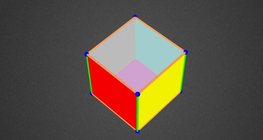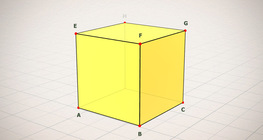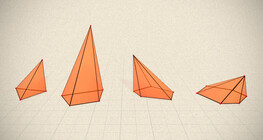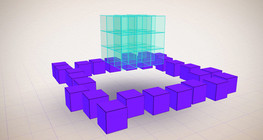Quantity: 0

Total: 0,00

0

# Cuboid (exercises)

### Cuboid (exercises)

Edges, diagonals and faces of a cuboid can be identified by its vertices.

Mathematics

Keywords

cuboid, face diagonal, space diagonal, face, edge, vertex, exercise, game, mathematics

### Related items#### Cuboid

A cuboid is a polyhedron with six rectangular faces.#### Grouping of cuboids

This animation demonstrates various types of cuboids through everyday objects.#### Colouring a cube

Colouring the vertices, edges and faces of a given cube according to the criteria specified in the exercise.#### Cube (exercises)

Edges, diagonals and faces of a cube can be identified by its vertices.#### Cube puzzle

Building cubes shown in several views from the available unit cubes aids spatial vision and other skills.#### Cube sections (exercise)

Examining solids formed by the intersection of a cube and a plane.#### Net of a cube (exercises)

Not all nets consisting of 6 congruent squares are foldable into cubes.#### Regular square pyramid

A regular square pyramid is a right pyramid with a square base and four triangular faces.#### Building shapes (multi colour)

Build 3D shapes from unit cubes with the help of several views.#### Building shapes (one colour)

Build 3D shapes from unit cubes with the help of several views.#### Constructing shapes (3D)

Building 3D shapes into a given cube mesh with the help of several views.